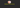# Data structures: How to implement Stacks and Queues in JavaScriptIn this tutorial, we are going to learn about stacks, queues datastructure and its implementation in javascript.

## What is a Stack?

A stack is a Collection of elements with operations push and pop. The name “Stack” comes from physical items placing the top on each other.

Stacks uses the Lifo principle (Last in First out) it means the last item we pushed into the stack removed first from the stack.

## Where stacks are used?

• Call stack
• Backtracking
• Browser History

Let’s Implement a stack by using a Singly Linked List.

We need to declare a class Node with two properties `data` and `next`

``````class Node{

constructor(data){
this.data = data;
this.next = null;
}

}``````

## Push Method

• It helps us to add the new elements to the stack.

In below code, we declared a Class Stack with three properties.

first: First element in the stack.

last: Last element in the stack.

size: size of the stack.

## push method pseudocode

1. create a method which accepts data as an argument.
2. Declare a new node with that data.
3. If a stack is empty then set first and last to the newly created node.
4. if there is a data in the stack then set new node.next to first property.
5. Update the first property with the new node.
6. Increment the stack size by 1.
``````class Stack{

constructor(){
this.first = null;
this.last = null
this.size = 0
}

push(data){

let node = new Node(data) // new node
// stack is empty
if(!this.first){
this.first = node
this.last = node
}else{
node.next = this.first
this.first = node
}

this.size++
}

}``````

## Pop method

• It helps us to remove the first element from the stack.

Pseudocode.

1. Create a new method called pop.
2. if the stack is empty return null.
3. declare a new variable called node and initialize with first property.
4. if there is more than one element in the stack then update the node variable with node.next and reset the first property to the updated node variable.
5. if there is one element in the stack then set the first and last property to null.
6. Decrement the size by 1.
`````` pop(){

if(!this.first) return null;

let node = this.first

if(node.next){
node = node.next
this.first = node
}else{
this.first = null
this.last = null
}

this.size--
}``````

## Time complexity

Adding and removing elements from the stack takes constant time O(1)

Tests

## Queues

What is a Queue?

A queue is a collection of items using the FIFO principle (First in First out) the first element we pushed into the queue is going to remove first.

Enqueue: Adding the elements at the end of the queue is called enqueue.

Dequeue Removing the elements from the first is called dequeue.

## Enqueue Method

Pseudo code.

1. create a enqueue method which accepts data as a first argument.
2. declare and initialize a node variable with the data.
3. if the queue is empty then set a first and last property to new node.
4. if there is a data in the queue then set this.last.next property and last property to new node.
5. Increment the size by 1.
``````class Queue{
constructor(){
this.first = null;
this.last = null
this.size = 0
}

enqueue(data){

let node = new Node(data) // new node
//queue is empty
if(!this.first){
this.first = node
this.last = node
}else{
this.last.next = node
this.last = node
}
// incrementing queue size
this.size++
}``````

## Dequeue Method

Pseudocode.

1. Create a new method called dequeue.
2. if the Queue is empty return null.
3. declare a new variable called node and initialize with first property.
4. if there is more than one element in the Queue then update the node variable with node.next and reset the first property to the updated node variable.
5. if there is one element in the Queue then set the first and last property to null.
6. Decrement the size by 1.
`````` dequeue(){

if(!this.first) return null;

let node = this.first

if(node.next){
node = node.next
this.first = node
}else{
this.first = null
this.last = null
}

this.size--
}``````

## Time complexity

Adding and removing elements from the Queue takes constant time O(1).

Happy coding…

## Top Udemy Courses##### JavaScript - The Complete Guide 2020 (Beginner + Advanced)
45,614 students enrolled
52 hours of video content
View Course##### React - The Complete Guide (incl Hooks, React Router, Redux)
284,472 students enrolled
40 hours of video content
View Course##### Vue - The Complete Guide (w/ Router, Vuex, Composition API)
130,921 students enrolled
48.5 hours of video content
View Course